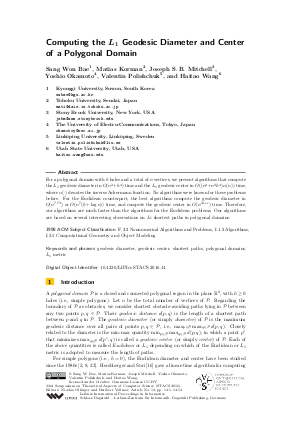Document# Computing the L1 Geodesic Diameter and Center of a Polygonal Domain

### Authors Sang Won Bae, Matias Korman, Joseph S. B. Mitchell, Yoshio Okamoto, Valentin Polishchuk, Haitao Wang## File

LIPIcs.STACS.2016.14.pdf
• Filesize: 0.66 MB
• 14 pages

## Cite As

Sang Won Bae, Matias Korman, Joseph S. B. Mitchell, Yoshio Okamoto, Valentin Polishchuk, and Haitao Wang. Computing the L1 Geodesic Diameter and Center of a Polygonal Domain. In 33rd Symposium on Theoretical Aspects of Computer Science (STACS 2016). Leibniz International Proceedings in Informatics (LIPIcs), Volume 47, pp. 14:1-14:14, Schloss Dagstuhl - Leibniz-Zentrum für Informatik (2016)
https://doi.org/10.4230/LIPIcs.STACS.2016.14

## Abstract

For a polygonal domain with h holes and a total of n vertices, we present algorithms that compute the L_1 geodesic diameter in O(n^2+h^4) time and the L_1 geodesic center in O((n^4+n^2 h^4)*alpha(n)) time, where alpha(.) denotes the inverse Ackermann function. No algorithms were known for these problems before. For the Euclidean counterpart, the best algorithms compute the geodesic diameter in O(n^{7.73}) or O(n^7(h+log(n))) time, and compute the geodesic center in O(n^{12+epsilon}) time. Therefore, our algorithms are much faster than the algorithms for the Euclidean problems. Our algorithms are based on several interesting observations on L_1 shortest paths in polygonal domains.
##### Keywords
• geodesic diameter
• geodesic center
• shortest paths
• polygonal domains
• L1 metric

## Metrics

• Access Statistics
• Total Accesses (updated on a weekly basis)
0

## References

1. H.-K. Ahn, L. Barba, P. Bose, J.-L. De Carufel, M. Korman, and E. Oh. A linear-time algorithm for the geodesic center of a simple polygon. In Proc. of the 31st Symposium on Computational Geometry (SoCG), pages 209-223, 2015.2. T. Asano and G. Toussaint. Computing the geodesic center of a simple polygon. Technical Report SOCS-85.32, McGill University, Montreal, Canada, 1985.3. S.W. Bae, M. Korman, J.S.B. Mitchell, Y. Okamoto, V. Polishchuk, and H. Wang. Computing the L₁ geodesic diameter and center of a polygonal domain. arXiv:1512.07160, 2015.4. S.W. Bae, M. Korman, and Y. Okamoto. The geodesic diameter of polygonal domains. Discrete and Computational Geometry, 50:306-329, 2013.5. S.W. Bae, M. Korman, and Y. Okamoto. Computing the geodesic centers of a polygonal domain. In Proc. of the 26th Canadian Conference on Computational Geometry, 2014.6. S.W. Bae, M. Korman, Y. Okamoto, and H. Wang. Computing the L₁ geodesic diameter and center of a simple polygon in linear time. Computational Geometry: Theory and Applications, 48:495-505, 2015.7. R. Bar-Yehuda and B. Chazelle. Triangulating disjoint Jordan chains. International Journal of Computational Geometry and Applications, 4(4):475-481, 1994.8. B. Chazelle. A theorem on polygon cutting with applications. In Proc. of the 23rd Annual Symposium on Foundations of Computer Science, pages 339-349, 1982.9. D.Z. Chen and H. Wang. A nearly optimal algorithm for finding L₁ shortest paths among polygonal obstacles in the plane. In Proc. of the 19th European Symposium on Algorithms, pages 481-492, 2011.10. D.Z. Chen and H. Wang. Computing the visibility polygon of an island in a polygonal domain. In Proc. of the 39th International Colloquium on Automata, Languages and Programming, pages 218-229, 2012. Journal version published online in Algorithmica, 2015.11. D.Z. Chen and H. Wang. L₁ shortest path queries among polygonal obstacles in the plane. In Proc. of the 30th Symposium on Theoretical Aspects of Computer Science, pages 293-304, 2013.12. D.Z. Chen and H. Wang. Visibility and ray shooting queries in polygonal domains. Computational Geometry: Theory and Applications, 48:31-41, 2015.13. H. Edelsbrunner, L.J. Guibas, and M. Sharir. The upper envelope of piecewise linear functions: Algorithms and applications. Discrete and Computational Geometry, 4:311-336, 1989.14. L.J. Guibas, J. Hershberger, D. Leven, M. Sharir, and R.E. Tarjan. Linear-time algorithms for visibility and shortest path problems inside triangulated simple polygons. Algorithmica, 2(1-4):209-233, 1987.15. J. Hershberger and J. Snoeyink. Computing minimum length paths of a given homotopy class. Computational Geometry: Theory and Applications, 4(2):63-97, 1994.16. J. Hershberger and S. Suri. Matrix searching with the shortest-path metric. SIAM Journal on Computing, 26(6):1612-1634, 1997.17. R. Inkulu and S. Kapoor. Planar rectilinear shortest path computation using corridors. Computational Geometry: Theory and Applications, 42(9):873-884, 2009.18. S. Kapoor, S.N. Maheshwari, and J.S.B. Mitchell. An efficient algorithm for Euclidean shortest paths among polygonal obstacles in the plane. Discrete and Computational Geometry, 18(4):377-383, 1997.19. J.S.B. Mitchell. An optimal algorithm for shortest rectilinear paths among obstacles. In the 1st Canadian Conference on Computational Geometry, 1989.20. J.S.B. Mitchell. L₁ shortest paths among polygonal obstacles in the plane. Algorithmica, 8(1):55-88, 1992.21. R. Pollack, M. Sharir, and G. Rote. Computing the geodesic center of a simple polygon. Discrete and Computational Geometry, 4(1):611-626, 1989.22. S. Schuierer. Computing the L₁-diameter and center of a simple rectilinear polygon. In Proc. of the International Conference on Computing and Information, pages 214-229, 1994.23. S. Suri. Computing geodesic furthest neighbors in simple polygons. Journal of Computer and System Sciences, 39:220-235, 1989.X

Feedback for Dagstuhl Publishing